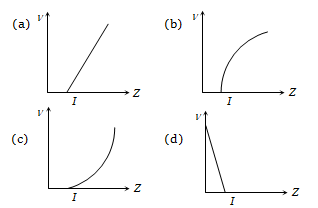The energy of a photon of light with wavelength 5000 Å is approximately 2.5 eV. This way the energy of an X-ray photon with wavelength 1Å would be
(a) 2.5/5000 eV             (b)
(c)            (d)

Concept Questions :-

X-ray
High Yielding Test Series + Question Bank - NEET 2020

Difficulty Level:

The graph that correctly represents the relation of frequency v of a particular characteristic X-ray with the atomic number Z of the material isConcept Questions :-

X-ray
High Yielding Test Series + Question Bank - NEET 2020

Difficulty Level:

If in nature there may not be an element for which the principal quantum number n > 4, then the total possible number of elements will be

(a) 60                    (b) 32
(c) 4                      (d) 64

Concept Questions :-

Various atomic model
High Yielding Test Series + Question Bank - NEET 2020

Difficulty Level:

In the Bohr's hydrogen atom model, the radius of the stationary orbit is directly proportional to (n = principle quantum number)
(a) ${\mathrm{n}}^{-1}$              (b) n
(c) ${\mathrm{n}}^{-2}$              (d) ${\mathrm{n}}^{2}$

Concept Questions :-

Bohr's model of atom
High Yielding Test Series + Question Bank - NEET 2020

Difficulty Level:

In the nth orbit, the energy of an electron ${\mathrm{E}}_{\mathrm{n}}=-\frac{13.6}{{\mathrm{n}}^{2}}\mathrm{eV}$ for hydrogen atom. The energy required to take the electron from first orbit to second orbit will be
(a) 10.2 eV              (b) 12.1 eV
(c) 13.6 eV              (d) 3.4 eV

Concept Questions :-

Bohr's model of atom
High Yielding Test Series + Question Bank - NEET 2020

Difficulty Level:

In the following atoms and moleculates for the transition from n= 2 to n = 1, the spectral line of minimum wavelength will be produced by
(a) Hydrogen atom               (b) Deuterium atom
(c) Uni-ionized helium           (d) di-ionized lithium

Concept Questions :-

Spectral series
High Yielding Test Series + Question Bank - NEET 2020

Difficulty Level:

The Lyman series of hydrogen spectrum lies in the region

(a) Infrared                (b) Visible
(c) Ultraviolet             (d) X- rays

Concept Questions :-

Spectral series
High Yielding Test Series + Question Bank - NEET 2020

Difficulty Level:

The size of an atom is of the order of

(a) ${10}^{-8}$ m         (b)
(c)         (d)

Concept Questions :-

Various atomic model
High Yielding Test Series + Question Bank - NEET 2020

Difficulty Level:

Which one of the series of hydrogen spectrum is in the visible region

(a) Lyman series              (b) Balmer series
(c) Paschen series            (d) Bracket series

Concept Questions :-

Spectral series
High Yielding Test Series + Question Bank - NEET 2020

Difficulty Level:

The Rutherford $\mathrm{\alpha }$-particle experiment shows that most of the $\mathrm{\alpha }$-particles pass through almost unscattered while some are scattered through large angles. What information does it give about the structure of the atom

(a) Atom is hollow

(b) The whole mass of the atom is concentrated in a small centre called nucleus

(c) Nucleus is positively charged

(d) All the above

Concept Questions :-

Various atomic model